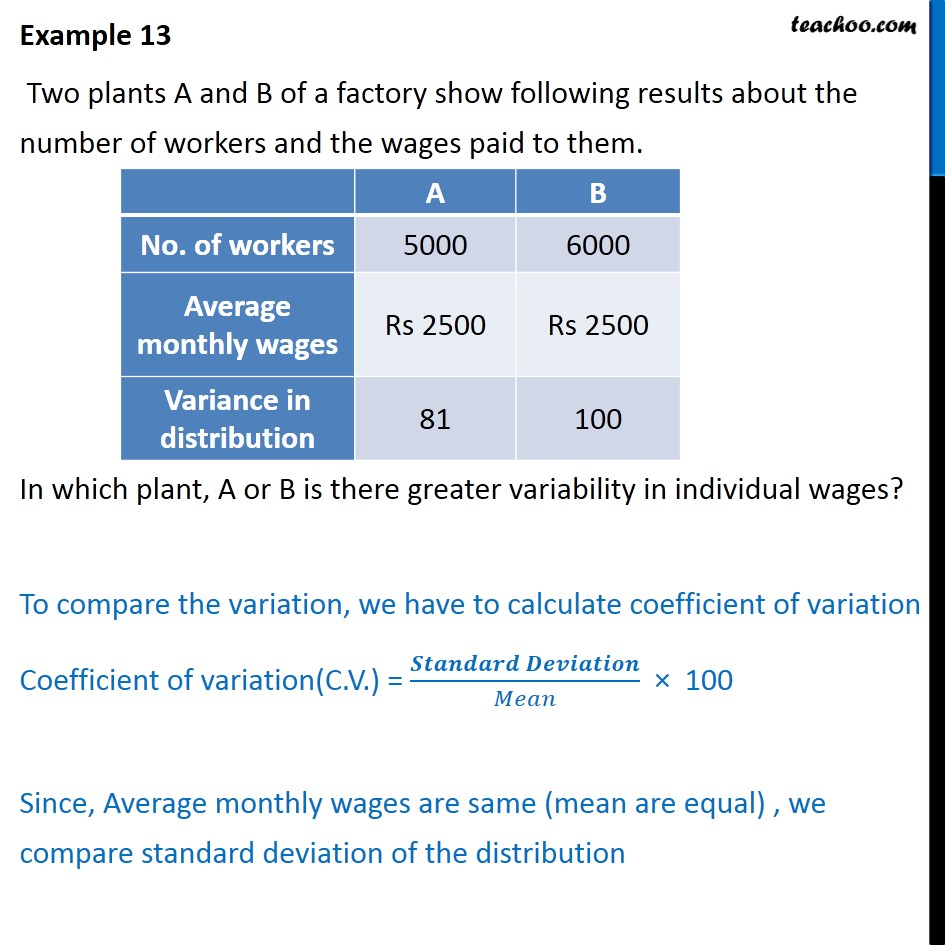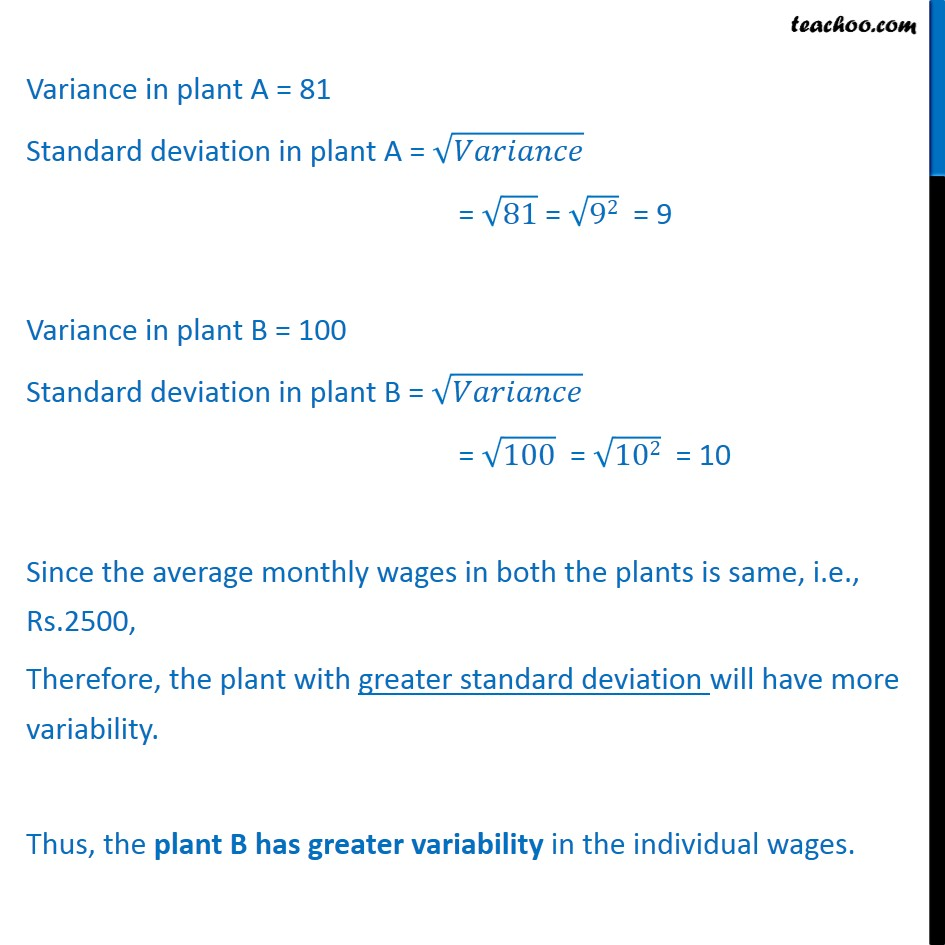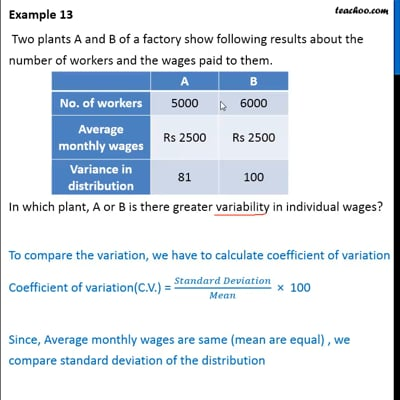Examples

Chapter 15 Class 11 Statistics (Term 1)
Serial order wiseThis video is only available for Teachoo black users

### Transcript

Example 13 Two plants A and B of a factory show following results about the number of workers and the wages paid to them. In which plant, A or B is there greater variability in individual wages? To compare the variation, we have to calculate coefficient of variation Coefficient of variation(C.V.) = 100 Since, Average monthly wages are same (mean are equal) , we compare standard deviation of the distribution Variance in plant A = 81 Standard deviation in plant A = = 81 = 92 = 9 Variance in plant B = 100 Standard deviation in plant B = = 100 = 102 = 10 Since the average monthly wages in both the plants is same, i.e., Rs.2500, Therefore, the plant with greater standard deviation will have more variability. Thus, the plant B has greater variability in the individual wages.